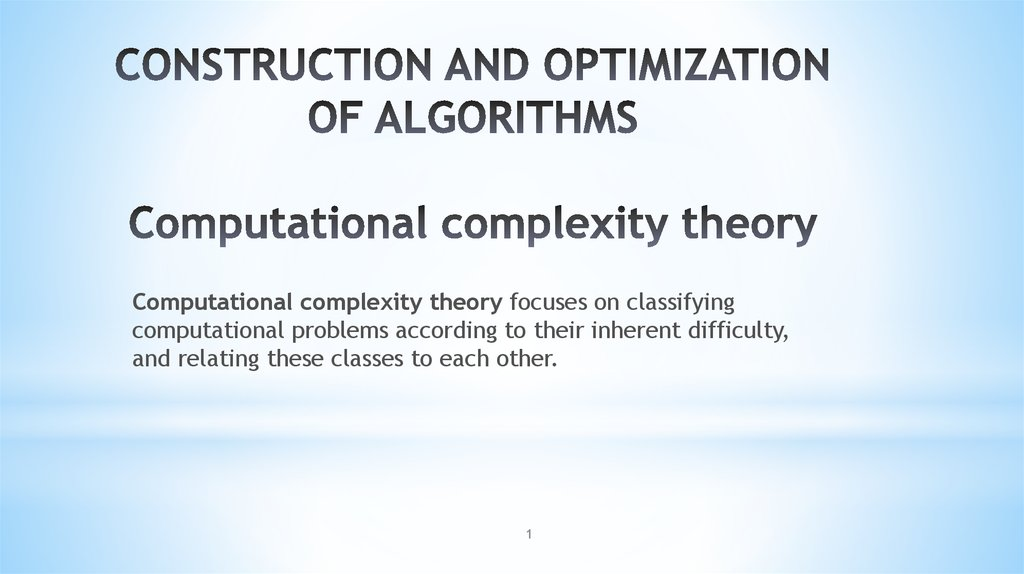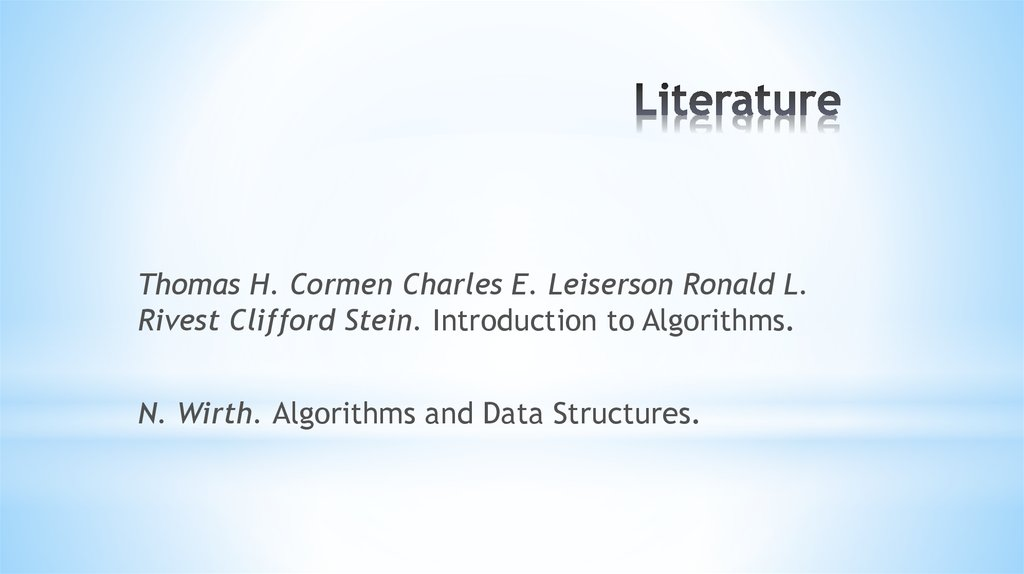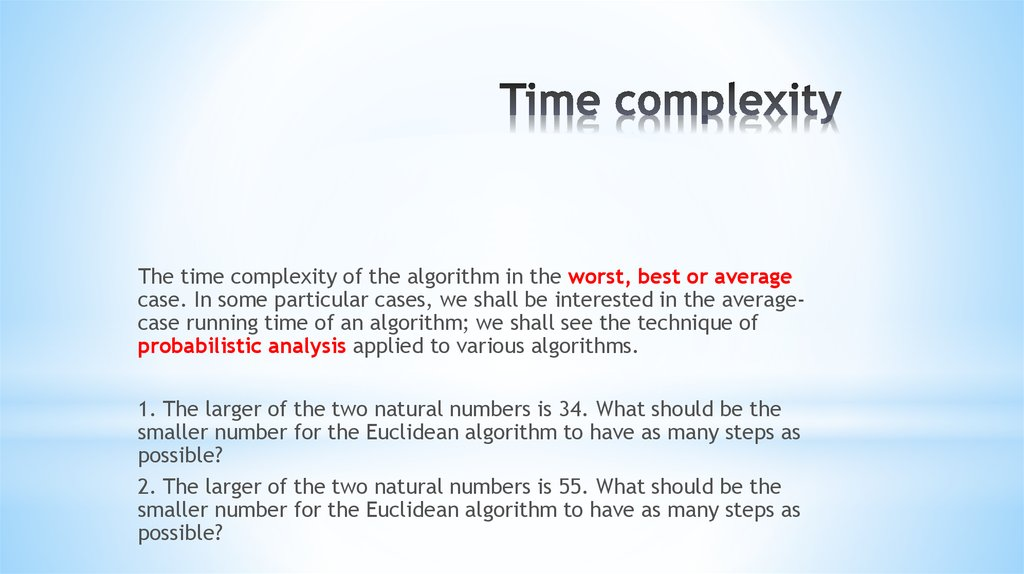# Construction and optimization of algorithms

## 1. CONSTRUCTION AND OPTIMIZATION OF ALGORITHMS Computational complexity theory

Computational complexity theory focuses on classifying
computational problems according to their inherent difficulty,
and relating these classes to each other.
1

## 2. Literature

Thomas H. Cormen Charles E. Leiserson Ronald L.
Rivest Clifford Stein. Introduction to Algorithms.
N. Wirth. Algorithms and Data Structures.

## 3. Time complexity

Тhe time complexity of the algorithm in the worst, best or average
case. In some particular cases, we shall be interested in the averagecase running time of an algorithm; we shall see the technique of
probabilistic analysis applied to various algorithms.
1. The larger of the two natural numbers is 34. What should be the
smaller number for the Euclidean algorithm to have as many steps as
possible?
2. The larger of the two natural numbers is 55. What should be the
smaller number for the Euclidean algorithm to have as many steps as
possible?

## 4. Asymptotic complexity

Examples - ?
Logarithmic, linear, polynomial, exponential complexity.

## 5. Other measures of complexity

are also used, such as
the amount of communication (used in communication
complexity),
the number of gates in a circuit (used in circuit complexity) and
the number of processors (used in parallel computing).
One of the roles of computational complexity theory is to
determine the practical limits on what computers can and cannot
do.

## 6. Other measures of complexity

If the created program is used only a few times, then the cost of
writing and debugging the program will dominate the total cost
of the program, that is, the actual execution time will not have a
significant impact on the total cost. In this case, you should
prefer the algorithm that is the easiest to realize.

## 7. Other measures of complexity

If the program will only work with “small” input data, the degree
of growth in the execution time will be less important than the
constant present in the asymptotic runtime formula. At the same
time, the notion of “smallness” of the input data depends on the
exact execution time of competing algorithms. There are
algorithms, such as the algorithm of integer multiplication, which
are asymptotically the most efficient, but which are never used in
practice even for large tasks, since their proportionality constants
far exceed those of other, simpler and less “efficient” algorithms.

## 8. Other measures of complexity

Sometimes there are incorrect algorithms that either get looped
or sometimes give the wrong result. But they still apply, because
in most cases they lead to the desired result. For example,
Kramer’s rule or resolution method.

## 14. Problems

1. Suppose weare comparing implementations of insertion sort
and merge sort on the same machine. For inputs of size n,
insertion sort runs in 8n2 steps, while merge sort runs in 64nlgn
steps. For which values of n does insertion sort beat merge sort?
2. What is the smallest value of n such that an algorithm whose
running time is 100n2 runs faster than an algorithm whose running
time is 2n on the same machine?

## 15. Problems

3. For each function f(n) and time t in the following table,
determine the largest size n of a problem that can be solved in
time t, assuming that the algorithm to solve the problem takes
f(n) microseconds.

## 16. Algorithms on graphs

First examples
1. Connect six points with non-intersecting segments so that 3 points
leave each point.
2. Connect 14 points with non-intersecting segments so that 4 points
leave each point.
3. Draw a closed six-pattern broken line that intersects each of its links
once.
4. The volleyball net has a size of 10 by 50 cells. What is the greatest
number of ropes can be cut so that the grid does not fall apart into
pieces?
16